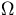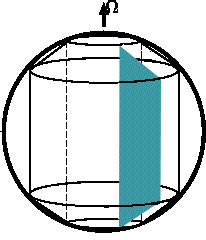We consider two-dimensional convective motion in a cylindrical annulus rotating with an angular velocityas shown in Figure 3. The cross section of the annulus is a trapezoid. The rotation axis is in the y direction. The gravity axis is always in the z direction. We consider two-dimensional motion in the x-z plane; the flow is assumed to be uniform in the y direction.Fig.3 A conceptual picture of the model